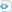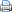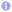# MPL – Data types

In earlier posts I gave an introduction to the Message Passing Library (MPL). With MPL processes can send and receive messages with data of different data types. What kind of data types are supported by MPL? MPL supports the same kinds of elementary data types as MPI does. These are:

• the integer types char, short int, int, long int, long long int as well as the unsigned variants thereof.
• the boolean type bool.
• the floating point types float, double and long double.
• the complex types std::complex<float>, std::complex<double>, std::complex<long double>.

The following program sends and receives data of each of these data types.

 Sending and receiving of data of elementary types.#include <cstdlib>
#include <complex>
#include <iostream>
#include <mpl/mpl.hpp>

int main() {
const mpl::communicator &comm_world=mpl::environment::comm_world();
if (comm_world.size()<2)
comm_world.abort(EXIT_FAILURE);
if (comm_world.rank()==0) {
// send a data item of each standard type
// note "char" might equal "signed char" or "unsigned char" depending on the compiler
char x1='A';                               comm_world.send(x1, 1);
signed char x2='B';                        comm_world.send(x2, 1);
unsigned char x3='C';                      comm_world.send(x3, 1);
signed short int x4=-1;                    comm_world.send(x4, 1);
unsigned short int x5=1;                   comm_world.send(x5, 1);
signed int x6=-10;                         comm_world.send(x6, 1);
unsigned int x7=10;                        comm_world.send(x7, 1);
signed long int x8=-100;                   comm_world.send(x8, 1);
unsigned long int x9=100;                  comm_world.send(x9, 1);
signed long long int x10=-1000;            comm_world.send(x10, 1);
unsigned long long int x11=1000;           comm_world.send(x11, 1);
bool x12=true;                             comm_world.send(x12, 1);
float x13=1.2345;                          comm_world.send(x13, 1);
double x14=2.3456;                         comm_world.send(x14, 1);
long double x15=3.4567;                    comm_world.send(x15, 1);
std::complex<float> x16(1.2, -1.2);        comm_world.send(x16, 1);
std::complex<double> x17(2.3, -2.3);       comm_world.send(x17, 1);
std::complex<long double> x18(3.4, -3.4);  comm_world.send(x18, 1);
}
if (comm_world.rank()==1) {
// receive a data item of each standard type
char x1;                        comm_world.recv(x1, 0);   std::cout << "x1 = " << x1 << '\n';
signed char x2;                 comm_world.recv(x2, 0);   std::cout << "x2 = " << x2 << '\n';
unsigned char x3;               comm_world.recv(x3, 0);   std::cout << "x3 = " << x3 << '\n';
signed short int x4;            comm_world.recv(x4, 0);   std::cout << "x4 = " << x4 << '\n';
unsigned short int x5;          comm_world.recv(x5, 0);   std::cout << "x5 = " << x5 << '\n';
signed int x6;                  comm_world.recv(x6, 0);   std::cout << "x6 = " << x6 << '\n';
unsigned int x7;                comm_world.recv(x7, 0);   std::cout << "x7 = " << x7 << '\n';
signed long int x8;             comm_world.recv(x8, 0);   std::cout << "x8 = " << x8 << '\n';
unsigned long int x9;           comm_world.recv(x9, 0);   std::cout << "x9 = " << x9 << '\n';
signed long long int x10;       comm_world.recv(x10, 0);  std::cout << "x10 = " << x10 << '\n';
unsigned long long int x11;     comm_world.recv(x11, 0);  std::cout << "x11 = " << x11 << '\n';
bool x12;                       comm_world.recv(x12, 0);  std::cout << "x12 = " << x12 << '\n';
float x13;                      comm_world.recv(x13, 0);  std::cout << "x13 = " << x13 << '\n';
double x14;                     comm_world.recv(x14, 0);  std::cout << "x14 = " << x14 << '\n';
long double x15;                comm_world.recv(x15, 0);  std::cout << "x15 = " << x15 << '\n';
std::complex<float> x16;        comm_world.recv(x16, 0);  std::cout << "x16 = " << x16 << '\n';
std::complex<double> x17;       comm_world.recv(x17, 0);  std::cout << "x17 = " << x17 << '\n';
std::complex<long double> x18;  comm_world.recv(x18, 0);  std::cout << "x18 = " << x18 << '\n';
}
return EXIT_SUCCESS;
}

MPL would not give a great advantage over MPI if it would only support these elementary data types as MPI does. However, MPL comes also with some support for user defined data types. To be able to exchange data of custom types via a message passing library. The message passing library must have some knowledge about the internal representation of user defined data types. Because C++ has very limited type introspection capabilities this knowledge cannot be obtained automatically by the message passing library. Usually information about the internal structure of user defined types (structures and classes) has to be exposed explicitly to the message passing library. Therefore, MPL supports message exchange of data where information about the internal representation can be obtained automatically and introduces a mechanism to expose the internal representation of custom types to MPL if this is not possible.

The data types, where MPL can infer their internal representation, are enumeration types, C arrays of constant size and the template classes std::array, std::pair and std::tuple of the C++ Standard Template Library. The only limitation is, that the C array and the mentioned template classes hold data elements of types that can be sent or received by MPL. This rule can be applied recursively, which allows to build quite complex data structures. For example, this means one can send and receive data of type std::pair<int, double>, because int and double can be sent or received. But also std::array<std::pair<int, double>, 8>, which represents 8 pairs of int and double, can be used. Enumeration types are internally dealt with like integers. Note that MPL determines automatically, which integer type is chosen by the compiler (or programmer) to represent an enumeration type. Examples are given below.

 Sending and receiving arrays, pairs and tuples.#include <cstdlib>
#include <complex>
#include <iostream>
#include <array>
#include <utility>
#include <tuple>
#include <mpl/mpl.hpp>

int main() {
const mpl::communicator &comm_world=mpl::environment::comm_world();
if (comm_world.size()<2)
comm_world.abort(EXIT_FAILURE);
const int N=8;
int x1[N];  // C array of constant size (known at compile time)
std::array<double, N> x2;  // STL array
std::pair<double, int> x3;  // STL pair
std::tuple<double, int, char> x4;  // STL tuple
if (comm_world.rank()==0) {
// send a C array of ints
for (int i=0; i<N; ++i)
x1[i]=i;
comm_world.send(x1, 1);
// send an STL array of doubles
for (int i=0; i<N; ++i)
x2[i]=i+0.01*i;
comm_world.send(x2, 1);
// send an STL pair of double and int
x3=std::make_pair(3.14, 77);
comm_world.send(x3, 1);
// send an STL tuple of double, int and char
x4=std::make_tuple(3.14, 77, 'Q');
comm_world.send(x4, 1);
}
if (comm_world.rank()==1) {
// recieve a C array of ints
comm_world.recv(x1, 0);
std::cout << "x1 = [";
for (auto i : x1)
std::cout << i << ", ";
std::cout << "]\n";
// receive an STL array of doubles
comm_world.recv(x2, 0);
std::cout << "x2 = [";
for (auto i : x2)
std::cout << i << ", ";
std::cout << "]\n";
// receive an STL pair of double and int
comm_world.recv(x3, 0);
std::cout << "x3 = [" << x3.first << ", " << x3.second << "]\n";
// receive an STL tuple of double, int  and char
comm_world.recv(x4, 0);
std::cout << "x4 = [" << std::get<0>(x4) << ", " << std::get<1>(x4) << ", " << std::get<2>(x4) << "]\n";
}
return EXIT_SUCCESS;
}
 Sending and receiving enumeration types.#include <cstdlib>
#include <complex>
#include <iostream>
#include <mpl/mpl.hpp>
#include <type_traits>

enum class colors : long long {red, green, blue=0x7fffffffffffffffll};

enum numbers_enum {one=1, two, three, four};

int main() {
const mpl::communicator &comm_world=mpl::environment::comm_world();
if (comm_world.size()<2)
comm_world.abort(EXIT_FAILURE);
if (comm_world.rank()==0) {
// send data items of enum types
colors c=colors::blue;  comm_world.send(c, 1);
numbers_enum n=three;   comm_world.send(n, 1);
}
if (comm_world.rank()==1) {
// receive data items of enum types
colors c;        comm_world.recv(c, 0);  std::cout << static_cast<long long>(c) << '\n';
numbers_enum n;  comm_world.recv(n, 0);  std::cout << static_cast<int>(n) << '\n';
}
return EXIT_SUCCESS;
}

User defined data structures come usually as structures or classes. Provided that these classes hold only non-static non-const data members of types, which MPL is able to send or receive, it is possible to expose these data members to MPL via  template specialization of the class struct_builder such that messages containing objects of these classes can be exchanged. Template specialization of the class struct_builder is illustrated in the following program. The specialized template has to derived from base_struct_builder and the internal data representation of the user defined class is exposed to MPL in the constructor.

 Sending and receiving classes.#include <cstdlib>
#include <vector>
#include <iostream>
#include <mpl/mpl.hpp>

// a class with some non-const non-static data members
class structure {
private:
double d;
int i;
public:
// constructor
template<typename... T>
structure(double d=0, T... i) : d(d), i{i...} {
}
friend std::ostream& operator<<(std::ostream& stream,
const structure &str) {
stream << "d = " << str.d << ", i = [";
for (int j=0; j<7; ++j)
stream << str.i[j] << ", ";
stream << str.i << "]";
return stream;
}
friend class mpl::struct_builder<structure>;
};

namespace mpl {

// expose internal data representation to MPL via template specialization
template<>
class struct_builder<structure> : public base_struct_builder<structure> {
struct_layout<structure> layout;
public:
struct_builder() {
structure str;
// register structure or class
layout.register_struct(str);
// register each non-const non-static data member
// MPL must be able to send and receive types of all data members
layout.register_element(str.d);
layout.register_element(str.i);
// finally, expose data layout to MPL
define_struct(layout);
}
};

}

int main() {
const mpl::communicator &comm_world=mpl::environment::comm_world();
if (comm_world.size()<2)
comm_world.abort(EXIT_FAILURE);
structure str;
// send a single structure
if (comm_world.rank()==0) {
str=structure(1.2, 1, 77, 8, 5, 2, 9, 6, 4);
comm_world.send(str, 1);
}
}